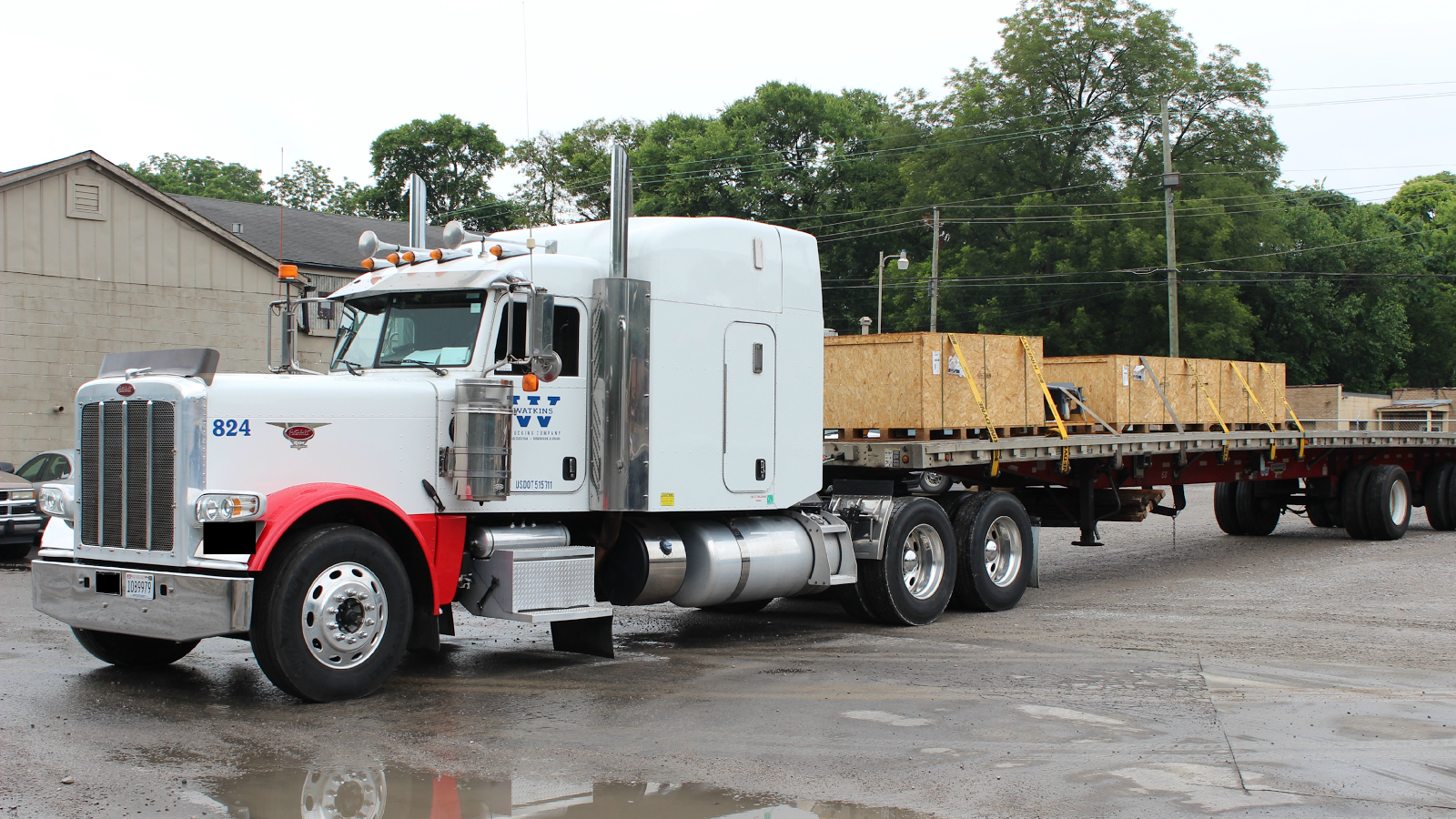## 20171003

### Physics quiz question: force of cargo load on truck magnitude

Physics 205A Quiz 3, fall semester 2017
Cuesta College, San Luis Obispo, CA"PACCAR Financial - Watkins Trucking 1"
TruckPR
flic.kr/p/oMJDhgA 600 kg load of cargo rests on the bed of a 8,850 kg stationary truck[*]. The magnitude of the normal force of the cargo load on the truck is:
(A) 0 N.
(B) 5.9×103 N.
(C) 8.67×104 N.
(D) 9.26×104 N.

[*] trucktrend.com/truck-reviews/163-0501-gm-kodiak-topkick-4x4/.

Correct answer (highlight to unhide): (B)

The cargo load has two forces acting on it:
Weight force of Earth on the cargo load (mcargo·g = 5.9×103 N, downwards).
Normal force of truck on the cargo load (upwards).
Because the cargo load is stationary, these two forces are equal in magnitude (each 5.9×103 N) and opposite in direction, due to Newton's first law, so the upwards normal force of truck on the cargo load must be 5.9×103 N in magnitude.

The truck has three forces acting on it:
Weight force of Earth on the truck (mtruck·g = 8.67×104 N, downwards).
Normal force of cargo load on the truck (downwards).
Normal force of ground on the truck (upwards).
Because the truck is stationary, the magnitudes of the two downward forces added together must equal the magnitude of the upwards force, due to Newton's first law. From Newton's third law, the downwards normal force of the cargo load on the truck must be equal in magnitude and opposite in direction to the upwards normal force of the truck on the cargo load, so they are each 5.9×103 N.

(Subsequently, the upwards normal force of the ground on the truck will be equal to the addition of the downwards force of the weight force of Earth on the truck (8.67×104 N) with the downwards normal force of the cargo load on the truck (5.9×103 N), and thus is 9.26×104 N.)

Sections 70854, 70855
Exam code: quiz03T4uC
(A) : 10 students
(B) : 35 students
(C) : 5 students
(D) : 4 students

Success level: 65%
Discrimination index (Aubrecht & Aubrecht, 1983): –0.06